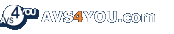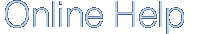Appendix
Appendix

# Frequency

Sampling frequency also impacts fidelity. The sampling frequency is essentially the number of times the sound event is quantized within a given time period. Sampling frequencies are specified in KiloHertz (KHz), a term meaning samples per second. The key in understanding how sampling frequency affects fidelity is the Nyquist sampling theorem. Basically, when applied to audio signals the Nyquist theorem states that the highest possible pitch in the sound is one-half that of the sampling frequency.

For example, "CD-quality" sound requires 16-bit words sampled at 44.1 KHz. Essentially this means 44,100 16-bit words (705,600 bits) are used to digitally describe each second of sound on a compact disc. The highest pitch possible is 22.05 KHz (approximately the top of human hearing range), which is half of 44.1 KHz.

•   File Format Settings
•   AVS Programs Codec Settings